7.5 Graphical method of solving a Quadratic equations:

We have learnt how to draw graph for an equation of type y=mx+c(Where m is a constant).

We have also observed that the equation of this type represents a straight line.

Let us learn to solve quadratic equation of type ax2 +bx+ c =0

We can solve the equation by two methods

First method:

ax2 +bx+ c = 0 can be written as

ax2= -bx-c

Let each be equal to y

So we have two equations y = ax2 and y =-bx-c

Draw the graph for both these equations. The intersecting points of the two graphs are solutions to the given equation ax2 +bx+ c=0

Note that y = =bx-c is an equation to a line. Problem 7.5.1 illustrates this method.

Second method:

Draw the graph ax2 +bx+ c and then find the points on the graph which touches x-axis (I.e. when y=0).The x-co-ordinates of the points on the graph, whose y-co-ordinates are zero, are roots of  the given equation. Problem 7.5.2 illustrates this method.

7.5 Problem 1:  Draw the graph for y=2x2 and y= 3+x and hence

1. Solve the equation 2x2-x-3=0

2. Find the value of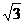Solution:

1. Solving of equation 2x2-x-3=0

Step 1: For few values of x tabulate the values of y (=2x2) as shown below:

 x à 0 1 -1 2 -2 y à 0 2 2 8 8 (x, y) (0,0) (1,2) (-1,2) (2,8) (-2,8)

Step 2: On a graph sheet mark the (x, y) co-ordinates. Join these points by a smooth curve. This smooth curve is called ‘parabola’

Step 3: For two values of x tabulate the values of y (=3+x) as shown below

 x à -1 1 y à 2 4 (x, y) (-1,2) (1,4)

Why did we ask you to find coordinates for only 2 values of x?

(y=3+x is of type y=mx+c and it represents a straight line. To draw a straight line, two points are enough)

Step 4: On a graph sheet mark these two (x,y) co-ordinates. Join these two points.

The parabola and the straight line cut each other at two points. They are (-1, 2) and (1.5, 4.5).

Their x co-ordinates are -1 and 1.5 respectively.

-1 and 3/2 satisfy the given equation 2x2-x-3=0.

Verification:

The given equation 2x2-x-3=0 is of the form ax2 +bx+ c =0. We have learnt that the roots of this equation arex = [-b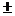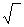(b2-4ac)]/2a      Here a=2, b= -1,c= -3(b2-4ac) =(1+24)=5

The roots are (15)/4 = -1 and 3/2 which are as derived using the graphical method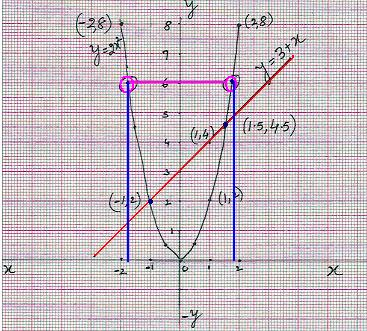2. Finding value of:

We need to find the value of y when x =. The graph for parabola is of the form y=2x2. Thus if x =then y=2x2= 6 So we need to find the value of x when y=6. From the graph we can see that there are two values of x (circled points in the graph in I Quadrant and II Quadrant) which satisfy y=6

We notice that x co-ordinate of the parabola at y=6 are   -1.7 and +1.7 which gives the value ofExercise and observations:

1. Draw few graphs for the equation y =mx2 for few values of m (both +ve and –ve) and observe the following:

-         All graphs are parabolas and pass through the origin

-         They are all symmetric about y-axis.

2. Draw few graphs for the equation x =my2 for few values of m (both +ve and –ve) and observe the following:

-         All graphs are parabolas and pass through the origin

-         They are all symmetric about x- axis.

7.5 Problem 2:  Solve the equation  2x2+3x-5=0

Solution:

Step 1: For few values of x tabulate the values of y(=2x2+3x-5) as given below:

 x à 0 1 -1 2 -2 -3 y à -5 0 -6 9 -3 4 (x, y) (0,-5) (1,0) (-1,-6) (2,9) (-2,-3) (-3,4)

Step 2: On a graph sheet mark these (x, y) co-ordinates.

Join these points by a smooth curve. This smooth curve is a parabola.

We need to find the point on graph when 2x2+3x-5=0( i,e when y=0)

We notice that the graph touches the x axis (note that y=0 for any point on x axis) at

x= -2.5(= -5/2) and at x=1.

Therefore 1 and -5/2 are the roots of the given equation.

Verification: The given equation 2x2+3x-5=0 is of the form ax2 +bx+ c =0

We have learnt that the roots of this equation arex = [-b(b2-4ac)]/2a    Here a=2, b= 3,c= -5Determinant b2-4ac = 9+40=49(b2-4ac) =7

The roots are (-37)/4

I.e. = 1 and -5/2 are the roots which we derived using the graphical method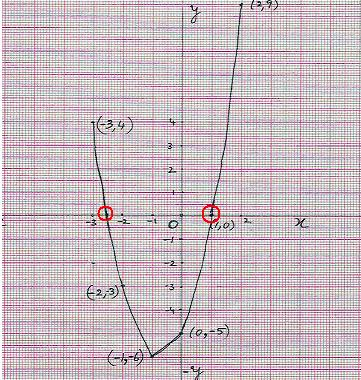Exercise and observations:

Draw graphs for the following equations in both the methods and observe the following

 Equation Method1 Method 2 Reason Draw 2 graphs Observations Draw 1 graph for Observations Determinant = b2-4ac = 1 2x2+2x-15=0 y = 2x2 y = -2x+15 The graphs meet at two points:(-5,0),(3,0) 2x2+2x-15 The graph touches x axis at 2 points and thus has two roots. 4-60=64 = 82 (perfect square) 2 4x2-4x+1=0 y = 4x2 y = 4x-1 The graphs meet at one point: (1/2,0) 4x2-4x+1=0 The graph touches x axis at 1  point and has only one root 16-16=0 (zero) 3 x2-6x+10=0 y = x2 y = 6x-10 The graphs do not meet at all! x2-6x+10=0 The graph does not touch x axis and thus no roots. 36-40 = -4 (negative)

7.5 Summary of learning

 No Points studied 1 Quadratic equations can be solved by drawing graphs